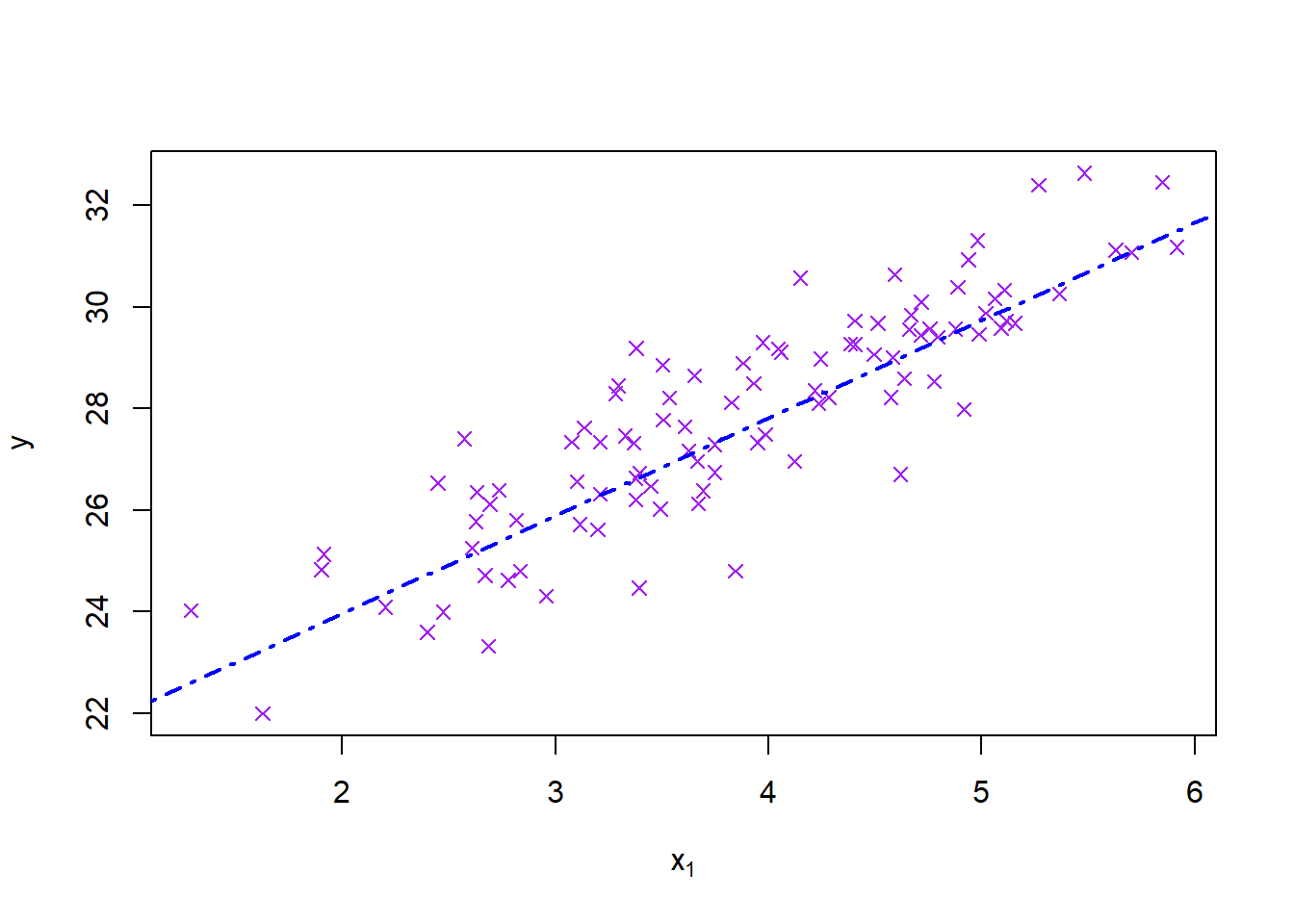Assignment Memo Example

Currently marking student 2001234567

# This code block (if run manually) generates an Excel file with different data for each student according to the list of student numbers

# In this case the data is for a regression with one significant explanatory variable and one irrelevant variable

library(openxlsx)
students <- c(2001234567,2012345678,2000000123)
nStudents <- length(students)
n <- 100
datasets <- vector('list',nStudents)
for (i in 1:nStudents) {
x1 <- rnorm(n,4,1)
x2 <- rgamma(n,4,2)
y <- 20 + 2*x1 + rnorm(n)
datasets[[i]] <- data.frame(y,x1,x2)
}
names(datasets) <- paste0('St',students)
write.xlsx(datasets, file = "Practical1data.xlsx")

Instructions

Your individual task with this assignment is to build a linear model and discuss it.

1. Read in the data from the sheet with your student number and verify that it was read in correctly.
2. Fit the standard linear regression model using main effects only, and give the summary.
3. Test the individual coefficients for significance if appropriate after testing the global hypothesis.
4. Add a scatterplot of $$y$$ versus $$x_1$$ with the regression line added.

The model is:

$y_i\sim N(\beta_0+\beta_1x_i,\ \sigma^2)$ ## Memorandum

library(openxlsx)
mydata <- read.xlsx('Practical1data.xlsx',paste0('St',params$st)) head(mydata) ## y x1 x2 ## 1 29.19206 3.378293 1.7129169 ## 2 28.64691 3.651103 1.4887481 ## 3 23.59713 2.398480 0.4670791 ## 4 29.88086 5.019538 3.5013878 ## 5 28.85903 3.502479 1.8389115 ## 6 26.96845 4.123522 2.5438280 || 1 mark for reading in their own data. 1 mark for checking it. || Q2: Linear model (s1 <- summary(model1 <- lm(y~x1+x2, data=mydata))) ## ## Call: ## lm(formula = y ~ x1 + x2, data = mydata) ## ## Residuals: ## Min 1Q Median 3Q Max ## -3.00376 -0.64894 -0.05359 0.80885 2.27539 ## ## Coefficients: ## Estimate Std. Error t value Pr(>|t|) ## (Intercept) 20.1353 0.4720 42.661 <2e-16 *** ## x1 1.9209 0.1077 17.844 <2e-16 *** ## x2 0.1705 0.1215 1.404 0.164 ## --- ## Signif. codes: 0 '***' 0.001 '**' 0.01 '*' 0.05 '.' 0.1 ' ' 1 ## ## Residual standard error: 1.07 on 97 degrees of freedom ## Multiple R-squared: 0.7689, Adjusted R-squared: 0.7641 ## F-statistic: 161.4 on 2 and 97 DF, p-value: < 2.2e-16 Q3: Model discussion In order to test the global hypothesis that no coefficients are significant we look at the F test p-value. If it is more than $$\alpha$$ we fail to reject the global null hypothesis and stop there. If the p-value is significant ($$<\alpha$$) then we test the individual hypotheses. || 2 marks for having a global conclusion, and discussing it. || The individual hypothesis is simply $$H_0:\beta_i=0\ vs\ H_1:\beta_i\ne 0$$. We reject the individual hypothesis for the significant terms based on the original p-values being less than $$\alpha$$ and conclude that these terms are useful and significant in predicting $$y$$. || 4 marks for discussing individual terms if appropriate. || Q4: Plot plot(mydata$x1, mydata\$y, pch=4, col='purple', main = '', xlab = expression(x), ylab = expression(y))
abline(coef(model1)[1:2], col='blue', lwd=2, lty=4)|| 2 marks for neat plot with line. ||

|| Total: 10 marks ||

Statistician